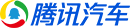# 腾讯汽车

## 全国城市列表

• 按拼音
• 按省份

<script type="text/javascript"> var theTable = document.getElementById("LIST_LM"); var spanPre = document.getElementById("spanPre"); var spanNext = document.getElementById("spanNext"); var nub = document.getElementById("shuzi"); var numberRowsInTable = theTable.getElementsByTagName("li").length;//总个数 var pageSize = 4;//一页多少个 var page = 1; function next(){ hideTable(); currentRow = pageSize * page; maxRow = currentRow + pageSize; if ( maxRow > numberRowsInTable ) maxRow = numberRowsInTable; for ( var i = currentRow; i< maxRow; i++ ){ theTable.getElementsByTagName("li")[i].style.display = ''; } page++; if ( maxRow == numberRowsInTable ) { nextText(); } preLink(); gg(); } function pre(){ hideTable(); page--; currentRow = pageSize * page; maxRow = currentRow - pageSize; if ( currentRow > numberRowsInTable ) currentRow = numberRowsInTable; for ( var i = maxRow; i< currentRow; i++ ){ theTable.getElementsByTagName("li")[i].style.display = ''; } if ( maxRow == 0 ){ preText(); } nextLink(); gg(); } //隐藏所有 function hideTable(){ for ( var i = 0; i<numberRowsInTable; i++ ){ theTable.getElementsByTagName("li")[i].style.display = 'none'; } } //总共页数 function pageCount(){ var count = 0; if ( numberRowsInTable%pageSize != 0 ) count = 1; return parseInt(numberRowsInTable/pageSize) + count; } //显示链接 function preLink(){ spanPre.innerHTML = "<a target=_self onclick='pre();' href='#blogtop'>上一页</a>";} function preText(){ spanPre.innerHTML = "<span class='na'>上一页</span>";} function nextLink(){ spanNext.innerHTML = "<a target=_self onclick='next();' href='#blogtop'>下一页</a>";} function nextText(){ spanNext.innerHTML = "<span class='na'>下一页</span>";} //隐藏其它页 function hide(){ for ( var i = pageSize; i<numberRowsInTable; i++ ){ theTable.getElementsByTagName("li")[i].style.display = 'none'; } nextLink(); } function pages(n){ hideTable(); page=n; if(n==1){ for ( var i = 0; i<pageSize; i++ ){ theTable.getElementsByTagName("li")[i].style.display = ''; } preText(); gg(); return false; } currentRow = pageSize * page; if(maxRow = currentRow + pageSize){ if ( maxRow > numberRowsInTable ) maxRow = numberRowsInTable; for ( var i = currentRow; i< maxRow; i++ ){ theTable.getElementsByTagName("li")[i].style.display = ''; } page++; if ( maxRow == numberRowsInTable ) nextText(); } if(maxRow = currentRow - pageSize){ hideTable(); page--; if ( currentRow > numberRowsInTable ) currentRow = numberRowsInTable; for ( var i = maxRow; i< currentRow; i++ ){ theTable.getElementsByTagName("li")[i].style.display = ''; } if ( maxRow == 0 ) preText(); } if(n==1){ preText(); nextLink(); } if(n==pageCount()){ nextText(); preLink(); } if(n!==1&&n!==pageCount()){ preLink(); nextLink(); } gg(); } function gg(){ nub.innerHTML=""; if(pageCount() ==1){preText();nextText();} if (page != 1) nub.innerHTML+= '<a target="_self" title="第一页" href="#blogtop" onclick="pages(1);">1</a>'; if (page>= 5) nub.innerHTML+='<span>...</span>'; if (pageCount() > page + 2) { var endPage = page + 2; } else { var endPage = pageCount(); } for (var i = page - 2; i <= endPage; i++) { if (i > 0) { if (i == page) { nub.innerHTML += '<span title="您正在浏本页" class="now">' + i + '</span>'; } else { if (i != 1 && i != pageCount()) { nub.innerHTML += '<a href="#blogtop" onclick="pages(' + i + ');">' + i + '</a>'; } } } } if (page + 3 < pageCount()) nub.innerHTML += '<span>...</span>'; if (page != pageCount()){ nub.innerHTML += '<a title="最后一页" href="#blogtop" onclick="pages(' + pageCount() + ');">' + pageCount() + '</a>'; nextLink(); } } hide(); gg(); </script>《腾讯任务》栏目介绍：定位于精品导购栏目，将为特定人群精选车型、量身推荐，以最全面的展现形式来帮众多准车主下定决心。
腾讯任务将以诙谐幽默的情景短剧为表现手法，完整的将各车型亮点逐一展示，准车主可根据不同使用需求，选择出最适合自己的"座驾"。

### 策划团队

 策划： 腾讯汽车车讯组 负责人： 张跃 制作： 张楠、付军、张跃、裴雷 视觉： 倪翔 其他： 李博、王天咨